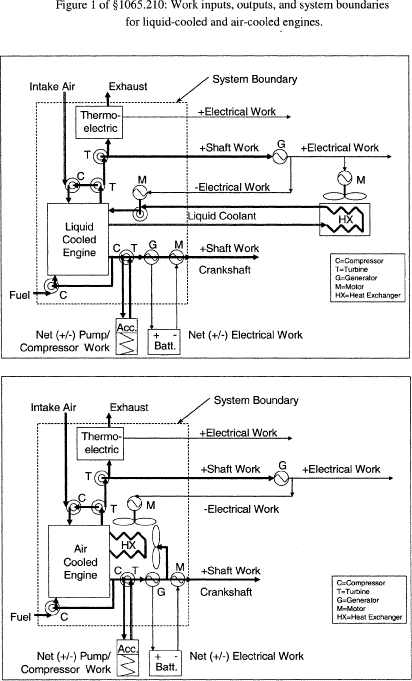# 40 CFR § 1065.210 - Work input and output sensors.

prev | next
§ 1065.210 Work input and output sensors.

(a)Application. Use instruments as specified in this section to measure work inputs and outputs during engine operation. We recommend that you use sensors, transducers, and meters that meet the specifications in Table 1 of § 1065.205. Note that your overall systems for measuring work inputs and outputs must meet the linearity verifications in § 1065.307. We recommend that you measure work inputs and outputs where they cross the system boundary as shown in Figure 1 of § 1065.210. The system boundary is different for air-cooled engines than for liquid-cooled engines. If you choose to measure work before or after a work conversion, relative to the system boundary, use good engineering judgment to estimate any work-conversion losses in a way that avoids overestimation of total work. For example, if it is impractical to instrument the shaft of an exhaust turbine generating electrical work, you may decide to measure its converted electrical work. As another example, you may decide to measure the tractive (i.e., electrical output) power of a locomotive, rather than the brake power of the locomotive engine. In these cases, divide the electrical work by accurate values of electrical generator efficiency (η<1), or assume an efficiency of 1 (η = 1), which would over-estimate brake-specific emissions. For the example of using locomotive tractive power with a generator efficiency of 1 (η = 1), this means using the tractive power as the brake power in emission calculations. Do not underestimate any work conversion efficiencies for any components outside the system boundary that do not return work into the system boundary. And do not overestimate any work conversion efficiencies for components outside the system boundary that do return work into the system boundary. In all cases, ensure that you are able to accurately demonstrate compliance with the applicable standards.(b)Shaft work. Use speed and torque transducer outputs to calculate total work according to § 1065.650.

(1)Speed. Use a magnetic or optical shaft-position detector with a resolution of at least 60 counts per revolution, in combination with a frequency counter that rejects common-mode noise.

(2)Torque. You may use a variety of methods to determine engine torque. As needed, and based on good engineering judgment, compensate for torque induced by the inertia of accelerating and decelerating components connected to the flywheel, such as the drive shaft and dynamometer rotor. Use any of the following methods to determine engine torque:

(i) Measure torque by mounting a strain gage or similar instrument in-line between the engine and dynamometer.

(ii) Measure torque by mounting a strain gage or similar instrument on a lever arm connected to the dynamometer housing.

(iii) Calculate torque from internal dynamometer signals, such as armature current, as long as you calibrate this measurement as described in § 1065.310.

(c)Electrical work. Use a watt-hour meter output to calculate total work according to § 1065.650. Use a watt-hour meter that outputs active power. Watt-hour meters typically combine a Wheatstone bridge voltmeter and a Hall-effect clamp-on ammeter into a single microprocessor-based instrument that analyzes and outputs several parameters, such as alternating or direct current voltage, current, power factor, apparent power, reactive power, and active power.

(d)Pump, compressor or turbine work. Use pressure transducer and flow-meter outputs to calculate total work according to § 1065.650. For flow meters, see §§ 1065.220 through 1065.248.

[70 FR 40516, July 13, 2005, as amended at 73 FR 37300, June 30, 2008; 79 FR 23760, Apr. 28, 2014]